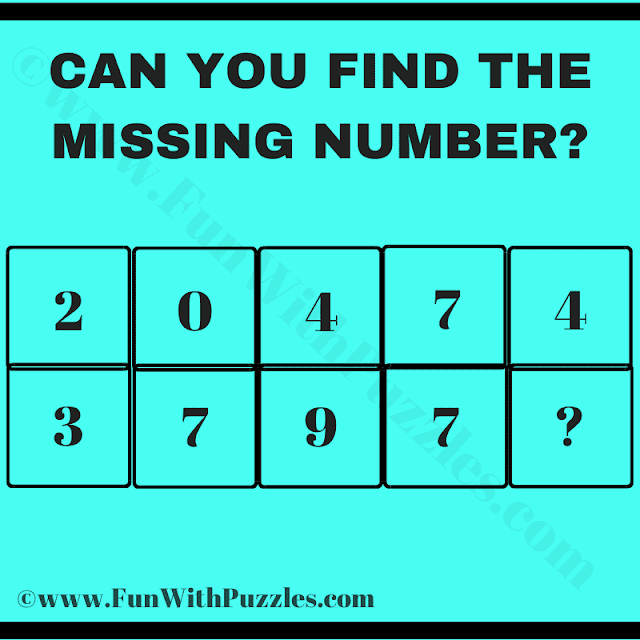This is one of the tricky logical reasoning mind puzzle questions which will break your brain. Solving these puzzles will first test your logical skills and then your mathematical skills. It means to solve this puzzle you require both good logical reasoning ability and good mathematical skills.
In this puzzle question, there are some digits in two rows. These digits relate to each other logically or mathematically. Your challenge in this mind puzzle is to crack the logical code and then mathematically find the value of the missing number in the last column.
Please write down your answer in the comments box along with your logical and mathematical reasoning used to solve this puzzle.Can you Find the Missing Number?

The answer to this "Mind Puzzle Question", can be viewed by clicking on the answer button.